# 再谈有序多分类的logistic回归：JMP操作及OR的再理解

1,375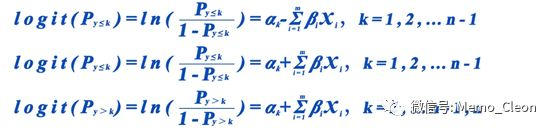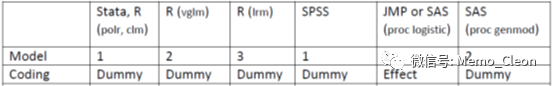另外自变量的编译方法和参照水平也不同，最终导致模型方程的参数估计值有所差异。When using statistical software, it is important to know: (1) what the default coding method is for categorical variable in regression models and (2) which level is being treated as the reference level. SAS, STATA, SPSS and R, for example, use dummy coding, whereas JMP uses effect coding by default. In SAS and SPSS by default, the last level (of the alphanumeric order) of the categorical variable is considered as the reference level; in STATA and R it is the first level. In JMP by default it is the last level which will be coded -1 for each effect variable.*2但是转化成相同的表达方式，结果应该是一致的。

JMP操作：示例同因变量有序多分类资料的logistic回归

1、数据录入：性别（Gender，数据类型：字符型；建模类型：名义型；列属性>值标签：0=男，1=女）；治疗方法（Treat，数据类型：字符型；建模类型：名义型；列属性>值标签：0=传统疗法，1=新型疗法）；疗效（effect，数据类型：数值型；建模类型：有序型；列属性>值标签：1=无效，2=有效，3=痊愈）；频数（fre，数据类型：数值型；建模类型：连续型）2、有序logistic回归：分析>>拟合模型

【选择角色变量】Y：effect；频数：fre

【构建效应模型】添加：Gender、Treat

【特质】有序型logistic

【运行】3、结果。增加wald检验和置信区间。不同于SPSS结果，JMP直接以报表的形式输出所有结果。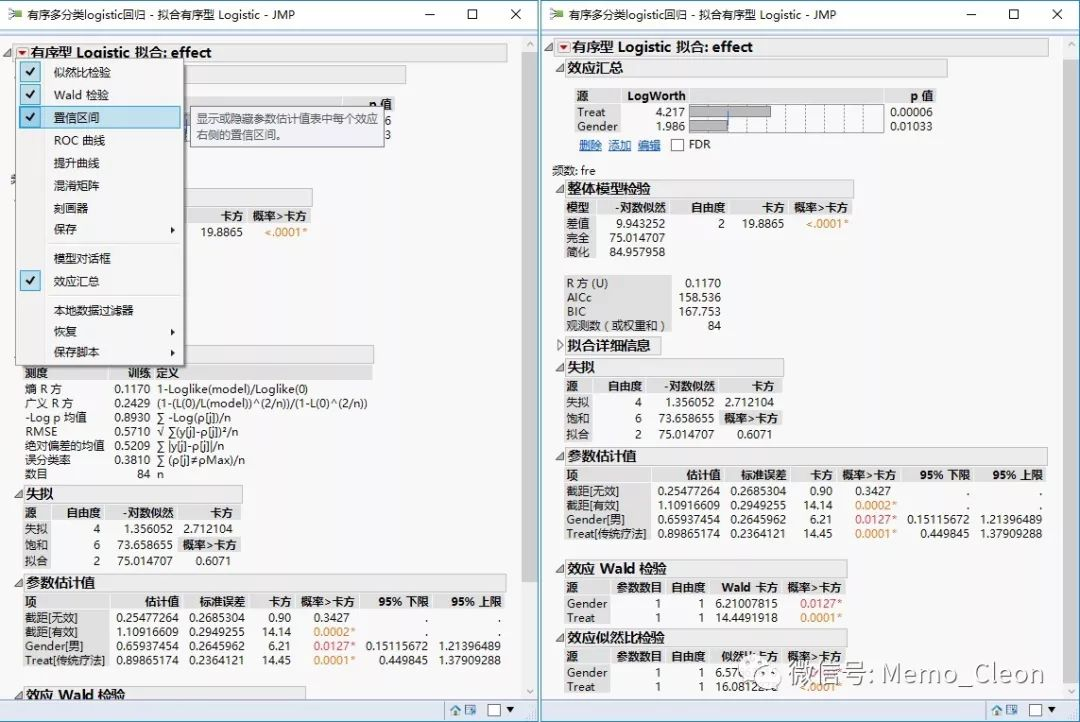4、结果解读*3

【1】效应汇总

• 源列出按 p 值升序排序的模型效应；

• logWorth显示每个模型效应的LogWorth，该值定义为-log10(p值)。该变换调整p值以提供适用于绘图的尺度。超过2的值在0.01水平下显著（-log10(0.01)=2）。选中FDR复选框可将logWorth列替换为FDR logWorth列；

• 条形图显示logWorth（或 FDR logWorth）值的条形图。该图在整数值处标绘垂直虚线，在值2处标绘一条蓝色参考线。

• P值显示每个模型效应的p值。这通常是模型结果的“效应检验”表或“效应似然比检验”表中显示的显著性检验所对应的p值。本例显示治疗方案和性别均有统计学意义。

【2】整体模型检验：指定模型是否显著好于简化模型（只含截距的模型），卡方统计量是拟合模型与仅包含截距的简化模型的负对数似然差值的两倍。仅包含截距的 –对数似然值84.96，完整模型减小到75.01，这个减小导致整体模型的似然比卡方统计量为9.94（自由度为2）。χ2=19.887，P<0.001，说明至少有一个自变量的偏回归系数不为0。

另外本部分结果还给出了R方、校正的 Akaike 信息准则 (AICc) 和 Bayesian 信息准则 (BIC) 。R方(U)即SPSS结果中的McFadden的伪R2。较小的AICc和BIC值表示拟合效果更好。

【3】拟合详细信息：对于熵R方和广义R方，值越接近1表示拟合效果越好。对于-log p均值、RMSE、绝对偏差的均值和误分类率，值越小表示拟合效果越好。

• 熵R方：等价R方(U)；

• 广义R：方亦称为 Nagelkerke或Craig和Uhler R2，可参见SPSS结果；

• -log p：均值-log(p) 的平均值，其中 p 是与发生的事件关联的拟合概率；

• RMSE：均方根误差，其中差值为响应和 p （实际发生事件的拟合概率）之间的差值；

• 绝对偏差的均值：响应和p（实际发生事件的拟合概率）的差值绝对值的平均值；

• 误分类率：具有最高拟合概率的响应类别不是观测到的类别的比率。

【4】失拟检验：即拟合优度检验，饱和模型是否显著好于指定的模型。P=0.607＞0.05，拟合优度较好。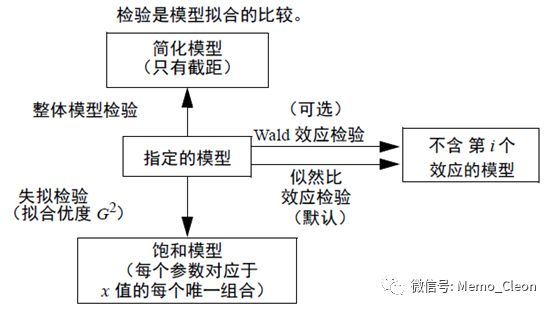【5】参数估计值。这是结果中最重要的部分，给出了截距和回顾系数、标准误、wald卡方检验及票%CI。最终获得两个模型回归方程如下：

• logit(Py≤1)=ln(Py≤1/(1- Py≤1))

= 0.255+0.659*Gender+0.899*Treat

• logit(Py≤2)=ln(Py≤2/(1- Py≤2))

= 1.109+0.659*Gender+0.899*Treat

• logit(Py≤1)=ln(Py≤1/(1- Py≤1))

=-1.303+1.319·Gender+1.797·Treat

• logit(Py≤2)=ln(Py≤2/(1- Py≤2))

=-0.449+1.319·Gender+1.797·Treat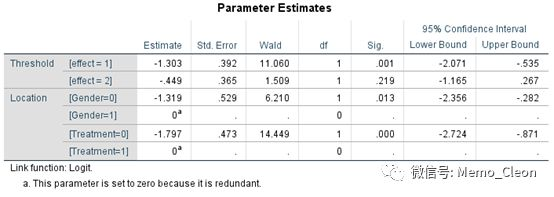SPSS的模型中回归方程的截距是自变量参照水平的均值。自变量采用的是哑变量编译（dummy coding）方式，当自变量为二分类时，两个变量的赋值分别是0和1。以性别为例，截距实际上就是固定自变量treatment，当Gender=0（男）时logit(P)值，即截距是参照组的logit(P)值。当Gender=1（女）时，logit(P)=截距+β。

Y1=logit(Px=0)=ln(Odds0)=α+βX(X=0)即ln(Odds0)=α

Y2= logit(Px=1)= ln(Odds1)=α+βX(X=1)即ln(Odds1)=α+β

∴ln(Odd0)-ln(Odd1)= α-(α+β)=-β 即ln(Odd0/Odd1)=-β 即lnOR=-β

OR=exp(-β)

JMP模型中回归方程的截距是自变量各水平均数的均值。自变量采用的是效果编译（effect coding）方式，当自变量为二分类时，第一个（Gender=0）和第二个水平（Gender=1）分别编码为1和-1。

Y1=logit(Px=1)=ln(Odds1)=α+βX(X=1)，即ln(Odds1)=α+β

Y2= logit(Px=-1)= ln(Odds-1)=α+βX(X=-1)，即ln(Odds-1)=α-β

∴ln(Odds1)-ln(Odds-1)=(α+β)-(α-β)=2β，即ln(Odd1/Odd-1)=2β，即lnOR=2β

OR=exp(2β)

Cornell University Statistical Consulting Unite的“Ordinal Logistic Regression Models and Statistical Software:What You Need to Know”一文中对不同的统计软件采用不同的模型以及回归系数的解释做了详细地、容易理解的介绍*1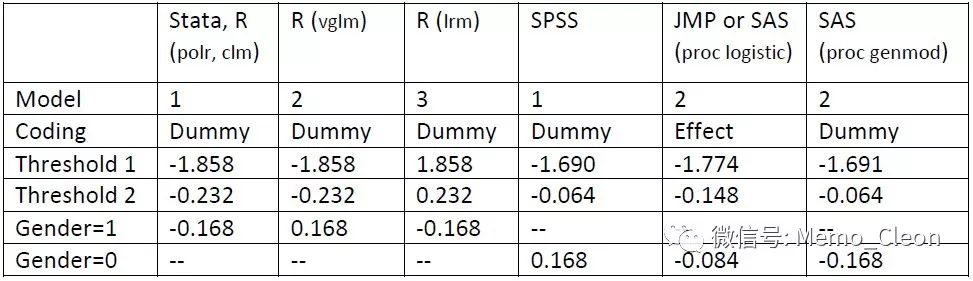【6】效应wald检验：回归系数的Wald卡方检验，结果同参数估计值。

【7】效应似然比检验。指定的模型是否显著好于不含给定效应的模型，同效应汇总。与不包含Gender和Treat的模型相比，最终模型均有统计学意义，说明含有Gender和Treat的最终模型拟合效果更好。

*1：Ordinal Logistic Regression Models and Statistical Software:What You Need to Know.

*2：Coding Categorical Variables in Regression Models:Dummy and Effect Coding.

*3：JMP:Fitting Linear Models.Second Edition.

END

• 版权声明 本文源自 一统浆糊 整理
• 转载请务必保留本文链接：https://www.plob.org/article/23473.html